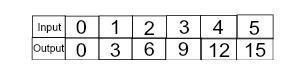# Function Table in Math: Definition, Rules & Examples

Instructions:

Choose an answer and hit 'next'. You will receive your score and answers at the end.

question 1 of 3

### If we represent our input by x and our output by y, then describe the rule that goes with this function table.Create Your Account To Take This Quiz

As a member, you'll also get unlimited access to over 75,000 lessons in math, English, science, history, and more. Plus, get practice tests, quizzes, and personalized coaching to help you succeed.

Try it risk-free for 30 days. Cancel anytime.

### 2. Which of the following function tables has rule y = 2x + 5 where x is an integer that is greater than or equal to -3 and less than or equal to 2?

Create your account to access this entire worksheet
A Premium account gives you access to all lesson, practice exams, quizzes & worksheets
Quizzes, practice exams & worksheets
Certificate of Completion
Create an account to get started

Using function tables is an important skill to have in mathematics, and this quiz/worksheet will help you assess your understanding of how to do so and let you put your knowledge to the test with practice problems.

## Quiz & Worksheet Goals

In these assessments you'll be tested on several applications and aspects of function tables including:

• Function table rules
• Creating function tables
• When it is appropriate to use function tables to describe functions

## Skills Practiced

This quiz and worksheet allow students to test the following skills:

• Problem solving - use acquired knowledge to solve practice problems involving the applications of function tables
• Distinguishing differences - ensure that you can differentiate between when it is and isn't appropriate to use function tables to describe functions
• Knowledge application - use your knowledge to create function tables

To learn more about function tables, review the accompanying lesson Function Table in Math: Definition, Rules & Examples. This lesson covers the following objectives:

• Define function
• Know the ways in which functions can be represented
• Know what is displayed by function tables
• Familiarize yourself with examples of function tables
• Know the rules of function tables
• Understand when to use a function table to describe a function
Final ExamACT Prep: Tutoring Solution
Status: Not Started
Chapter ExamACT Math - Functions: Tutoring Solution
Status: Not Started

Support